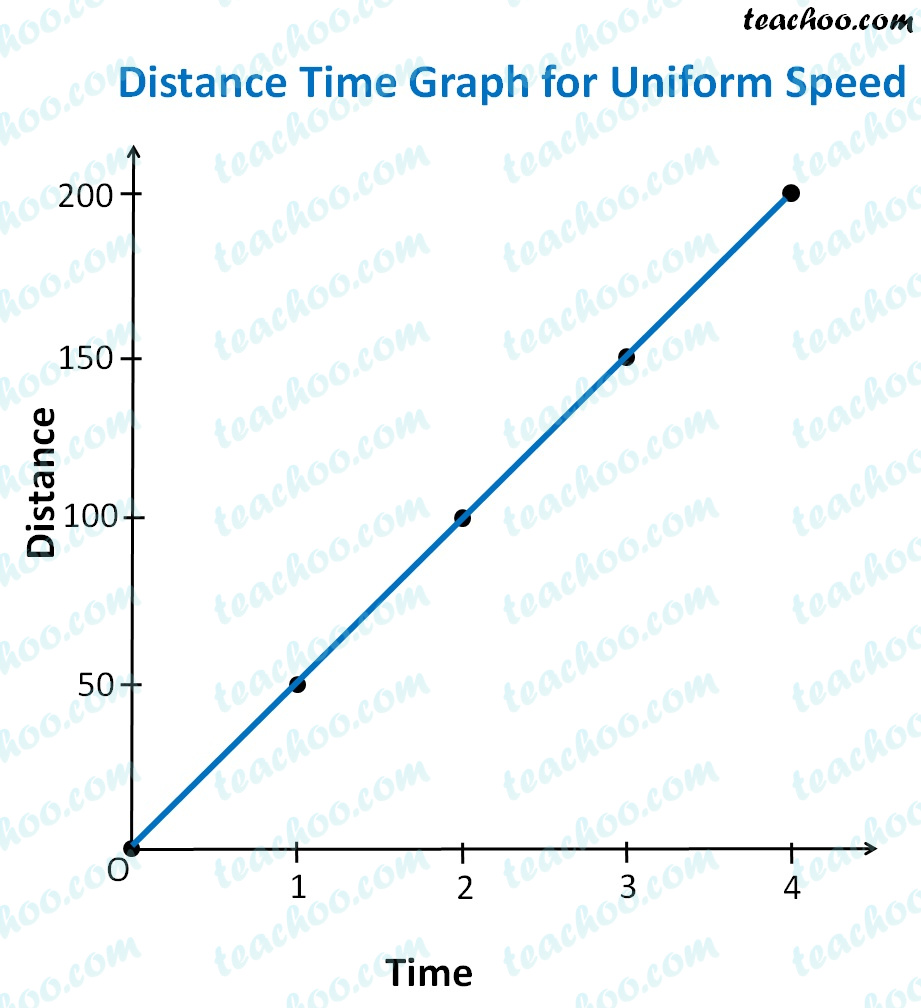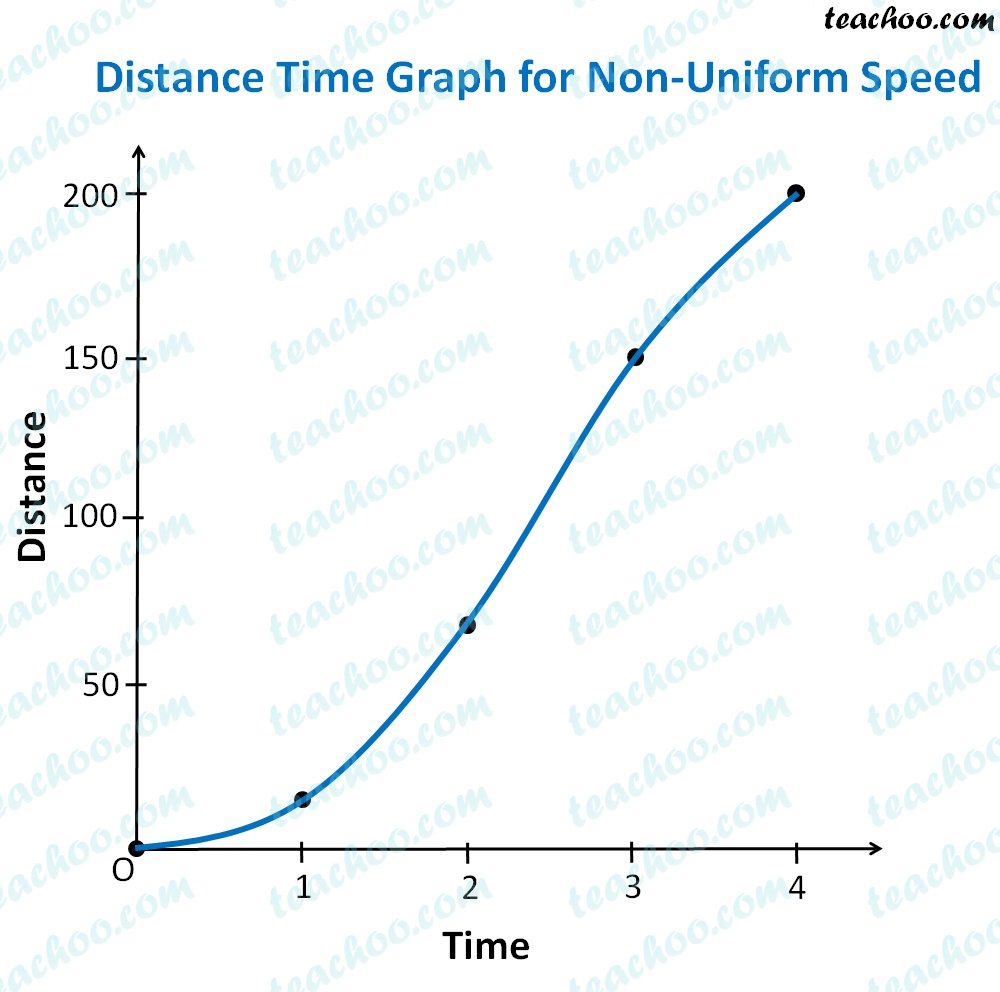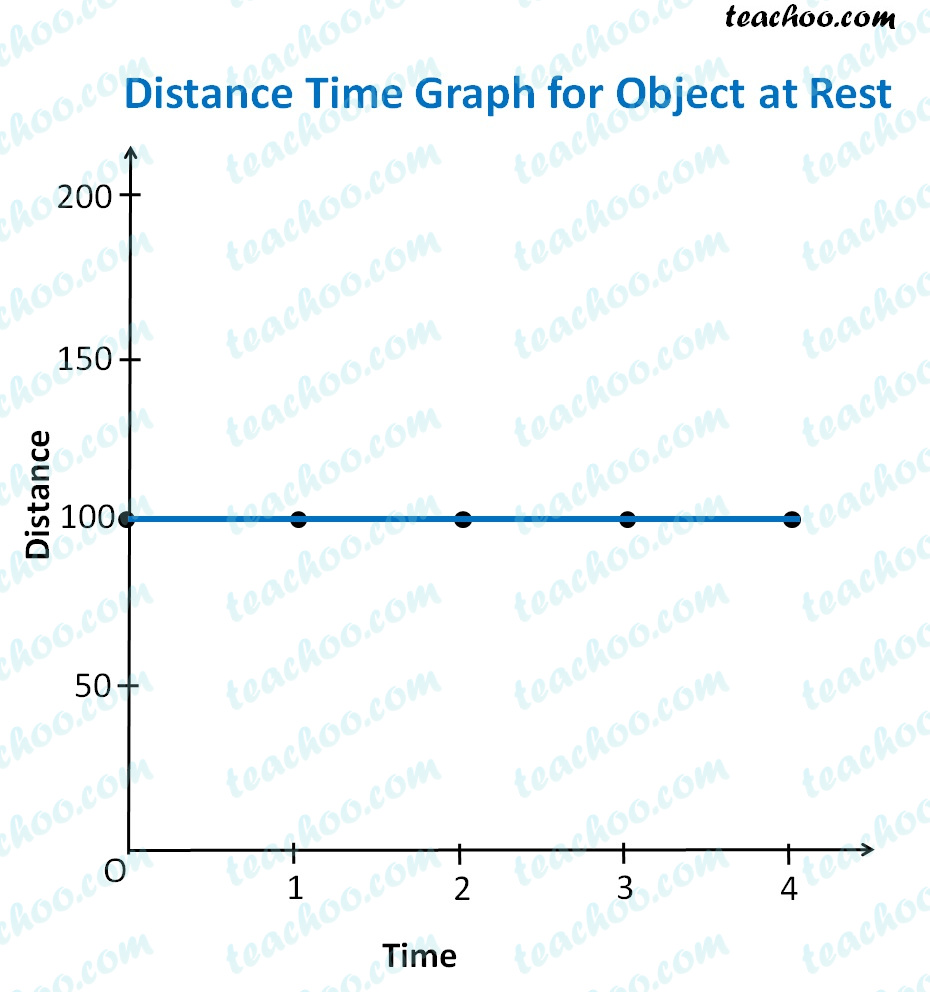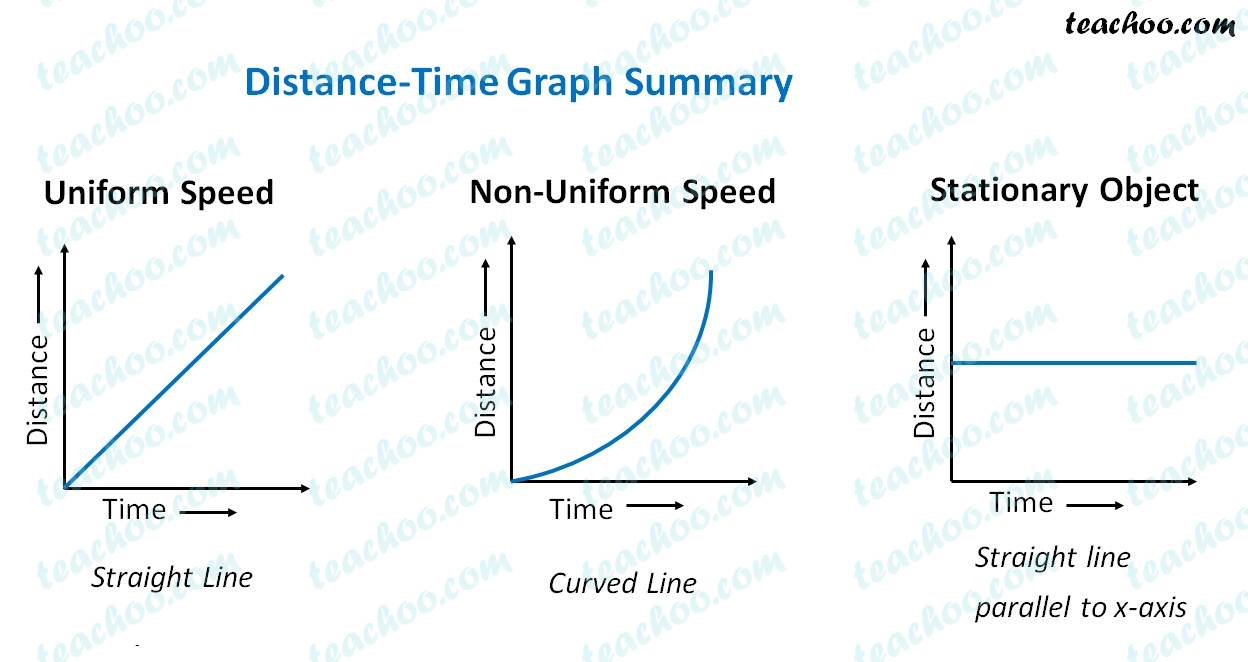Concepts

Class 9
Chapter 8 Class 9 - Motion

It is used to show Distance Travelled and Time Taken on a graph paper

Here,

Time Taken is shown on x axis

Distance is shown on y axis

## Distance Time Graph for Uniform Speed

Suppose an object travells at Uniform speed of 50 km/hour

It means

 Hour Distance Travelled 0 0 1 50 2 100 3 150 4 200

Plotting it in Graph

We show

Time on x axis

Distance on y axis## Distance Time Graph for Object Moving at Non Uniform Speed

Suppose an object travels at of 30 km/hour in first hour,50 km/hr in second hour,100 km in third hour ,140 km in fourth hour

It means

 Hour Distance Travelled 0 0 1 20 2 70 3 150 4 200## Distance Time Graph in case of Stationary Object

In case of Stationary object,Distance Travelled does not change

Suppose Distance travelled in 1 hour,2 hour ,3 hours remains fixed at 100 km

In this case Distance time graph will be as follows## Summary Of Distance Time Graph## Questions

NCERT Question 6 - Fig 8.11 shows the distance-time graph of three objects A,B and C. Study the graph and answer the following questions:

Learn in your speed, with individual attention - Teachoo Maths 1-on-1 Class